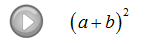Showing posts with label distribute. Show all posts
Showing posts with label distribute. Show all posts

## Sunday, November 4, 2012

### Multiplying Polynomials

The distributive property and the product rule for exponents are the keys to multiplication of polynomials. Remember the product rule,
It says that when we multiply two numbers with the same base we must add the exponents.

Monomial x Polynomial
Multiply.
A common mistake is to distribute when working with multiplication. The distributive property only applies when addition or subtraction separates the terms.
Binomial x Polynomial: Use the distributive property to multiply polynomials by binomials.
We can save a step by distributing each term in the binomial individually. Sometimes this technique is referred to as the FOIL method. (FOIL – Multiply the First, Outer, Inner and then Last terms together.)

Multiply.
When combining like terms, be sure that the variable parts are exactly the same. Go slow and work in an organized fashion because it is easy to make an error when many terms are involved.
It is good practice to recheck your distributive step.
Trinomial x Polynomial
The following problem is one that we will have to be able to do before moving on to the next algebra course. These are tedious, time consuming, and often worked incorrectly. Use caution, take your time and work slowly.
Function notation for multiplication looks like
Given the functions f and g find (f g)(x).
The following special products will simplify things if we memorize them.
This special product is often called difference of squares. Notice that the middle terms cancel because one term will always be positive and the other will be negative.
We may use these formulas as templates when multiplying binomials. It is a good idea to memorize them.

Multiply.